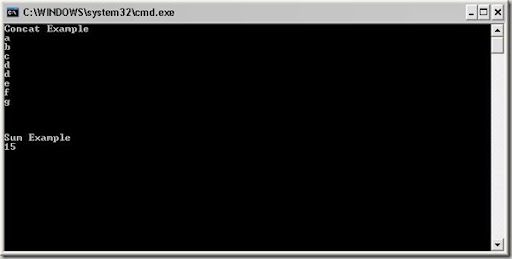Saturday, June 19, 2010

## Sum and Concat Operator operators in Linq.

Linq contains lots useful operators and i have found more two operators that can be help full in our day to day programming life. Here are explanation.

Concat Operator: Concat operator concats two entities into single entities its very use full when we are doing some string operator where need to do string operations like concatenation. Here in example i have taken two arrays and concat into single entity.

Sum Operator: When we are developing application like shopping cart and other stuff this operator can be use full where we need to calculate the total or order price and other stuff you can apply this operator array, list and anything that implemented IEnumerable interface. Here i have taken a integer array for the example.

Here is the code for both operators.

`namespace ConsoleApplication1{ class Program {     static void Main(string[] args)     {         string[] a = { "a", "b", "c", "d" };         string[] b = { "d","e","f","g"};               Console.WriteLine("Concat Example");         var CtResult = a.Concat<string>(b);         foreach (string s in CtResult)         {             Console.WriteLine(s);         }         int[] num = { 1, 2, 3, 4, 5 };         Console.WriteLine("\n\n\nSum Example");         var SumResult = num.Sum();         Console.WriteLine(SumResult);         Console.ReadKey();     } }}`
And here is the output of console application as expected.Technorati Tags: ,,,Share: